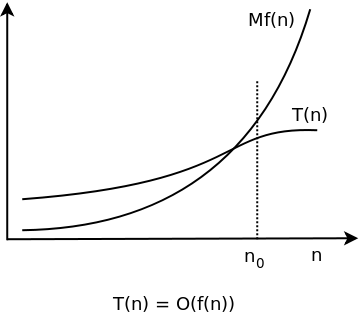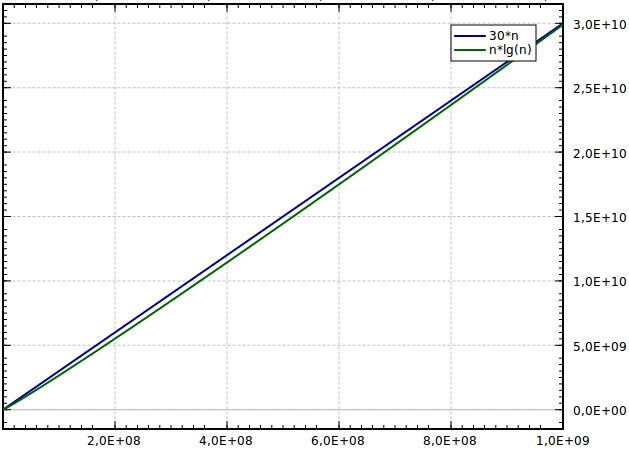# Big O notation: definition and examples

yourbasic.org

Big O notation is a convenient way to describe how fast a function is growing.## Definition

When we compute the time complexity T(n) of an algorithm we rarely get an exact result, just an estimate. That’s fine, in computer science we are typically only interested in how fast T(n) is growing as a function of the input size n.

For example, if an algorithm increments each number in a list of length n, we might say: “This algorithm runs in O(n) time and performs O(1) work for each element”.

Here is the formal mathematical definition of Big O.

Let T(n) and f(n) be two positive functions. We write T(n) ∊ O(f(n)), and say that T(n) has order of f(n), if there are positive constants M and n₀ such that T(n) ≤ M·f(n) for all n ≥ n₀.

This graph shows a situation where all of the conditions in the definition are met.In essence:

T(n) ∊ O(f(n)) means that T(n) doesn't grow faster than f(n).

## Constant time

According to the definition this means that there are constants M and n₀ such that T(n) ≤ M when n ≥ n₀. In other words, T(n) ∊ O(1) means that T(n) is smaller than some fixed constant, whose value isn’t stated, for all large enough values of n.

An algorithm with T(n) ∊ O(1) is said to have constant time complexity.

## Linear time

In the Time complexity article, we looked at an algorithm with complexity T(n) = n -1. Using Big O notation this can be written as T(n) ∊ O(n). (If we choose M = 1 and n₀ = 1, then T(n) = n - 1  ≤ 1·n when n ≥ 1.)

An algorithm with T(n) ∊ O(n) is said to have linear time complexity.

The second algorithm in the Time complexity article had time complexity T(n) = n2/2 - n/2. With Big O notation, this becomes T(n) ∊ O(n2), and we say that the algorithm has quadratic time complexity.

## Sloppy notation

The notation T(n) ∊ O(f(n)) can be used even when f(n) grows much faster than T(n). For example, we may write T(n) = n - 1 ∊ O(n2). This is indeed true, but not very useful.

## Ω and Θ notation

Big Omega is used to give a lower bound for the growth of a function. It’s defined in the same way as Big O, but with the inequality sign turned around:

Let T(n) and f(n) be two positive functions. We write T(n) ∊ Ω(f(n)), and say that T(n) is big omega of f(n), if there are positive constants m and n₀ such that T(n) ≥ m(f(n)) for all n ≥ n₀.

Big Theta is used to indicate that a function is bounded both from above and below.

T(n) ∊ Θ(f(n)) if T(n) is both O(f(n)) and Ω(f(n)).

### Example

T(n) = 3n3 + 2n + 7 ∊ Θ(n3)

• If n ≥ 1, then T(n) = 3n3 + 2n + 7 ≤ 3n3 + 2n3 + 7n3 = 12n3. Hence T(n) ∊ O(n3).
• On the other hand, T(n) = 3n3 + 2n + 7 > n3 for all positive n. Therefore T(n) ∊ Ω(n3).
• And consequently T(n) ∊ Θ(n3).

## Key takeaways

When analyzing algorithms you often come across the following time complexities.

Complexity
Θ(1) Good news
Θ(log n)
Θ(n)
Θ(n log n)
Θ(nk), where k ≥ 2 Bad news
Θ(kn), where k ≥ 2
Θ(n!)

### O(n log n) is really good

The first four complexities indicate an excellent algorithm. An algorithm with worst-case time complexity W(n) ∊ O(n log n) scales very well, since logarithms grow very slowly.

• log2 1,000 ≈ 10
• log2 1,000,000 ≈ 20
• log2 1,000,000,000 ≈ 30

In fact, Θ(n log n) time complexity is very close to linear – it takes roughly twice the time to solve a problem twice as big.n log n growth rate is close to linear

The last three complexities typically spell trouble. Algorithms with time complexity Ω(n2) are useful only for small input: n shouldn’t be more than a few thousand.

10,0002 = 100,000,000

An algorithm with quadratic time complexity scales poorly – if you increase the input size by a factor 10, the time increases by a factor 100.

### NextTime complexity of recursive functions

### Previous